## Sunday, March 13, 2011

I've accepted an offer from Google and will be joining their Tesseract team next week.

I first got interested in OCR when I faced a project at my previous job involving OCR of outdoor scenes and found it to be a very complex task, yet highly rewarding because it's easy to make incremental progress and see your learners working.

Current state-of-the-art OCR tools are not at human level of reading, partially because humans are able to take context into account. For instance, sentence below is unreadable if you don't assume English language.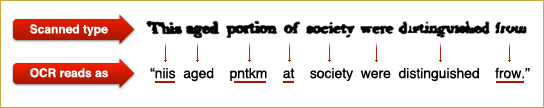Also, I'm excited to work on an open-source project. I'd like to live in a world where the best technologies are open-source and free to everyone.

## Saturday, March 05, 2011

### Linear Programming for Maximum Independent Set

Maximum independent set, or "maximum stable" set is one of classical NP-complete problems described in Richard Karp's 1972 paper "Reducibility Among Combinatorial Problems". Other NP-complete problems often have a simple reduction to it, for instance, p.3 of Tony Jebara's "MAP Estimation, Message Passing, and Perfect Graphs" shows how MAP inference in an arbitrary MRF reduces to Maximum Weight Independent Set.

The problem asks to find the largest set of vertices in a graph, such that no two vertices are adjacent.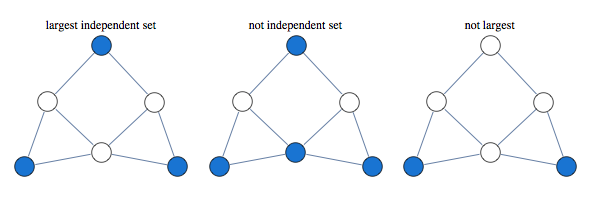Finding largest independent set is equivalent to finding largest clique in graph's complement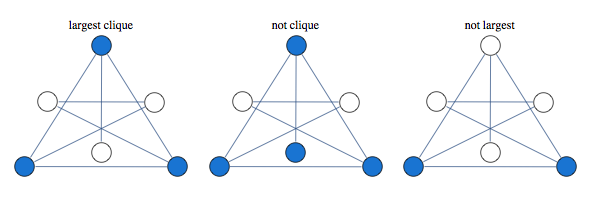A classical approach to solving this kind of problem is based on linear relaxation.

Take a variable for every vertex of the graph, let it be 1 if vertex is occupied and 0 if it is not occupied. We encode independence constraint by requiring that adjacent variables add up to 1. So we can consider the following graph and corresponding integer programming formulation: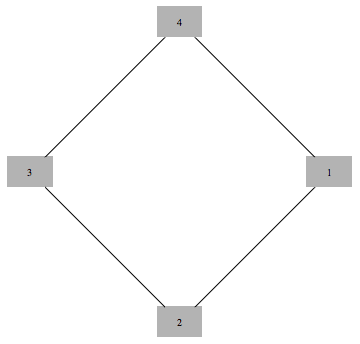$$\max x_1 + x_2 + x_3 + x_4$$

such that
$$\begin{array}{c} x_1+x_2 \le 1 \\\\ x_2+x_3 \le 1 \\\\ x_3+x_4 \le 1 \\\\ x_4+x_1 \le 1 \\\\ x_i\ge 0 \ \ \forall i \\\\ x_i \in \text{Integers}\ \forall i \end{array}$$

Solving this integer linear integer program is equivalent to the original problem of maximum independent set, with 1 value indicating that node is in the set. To get a tractable LP programme we drop the last constraint.

If we solve LP without integer constraints and get integer valued result, the result is guaranteed to be correct. In this case we say that LP is tight, or LP is integral.

Curiously, solution of this kind of LP is guaranteed to take only values 0,1 and $\frac{1}{2}$. This is known as "half-integrality". Integer valued variables are guaranteed to be correct, and we need to use some other method to determine assignments to half-integer variables.

For instance below is a graph for which first three variables get 1/2 in the solution of the LP, while the rest of the vertices get integral values which are guaranteed to be correct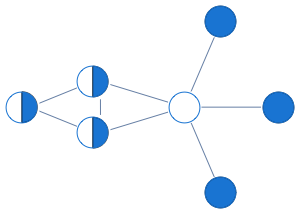Here's what this LP approach gives us for a sampling of graphs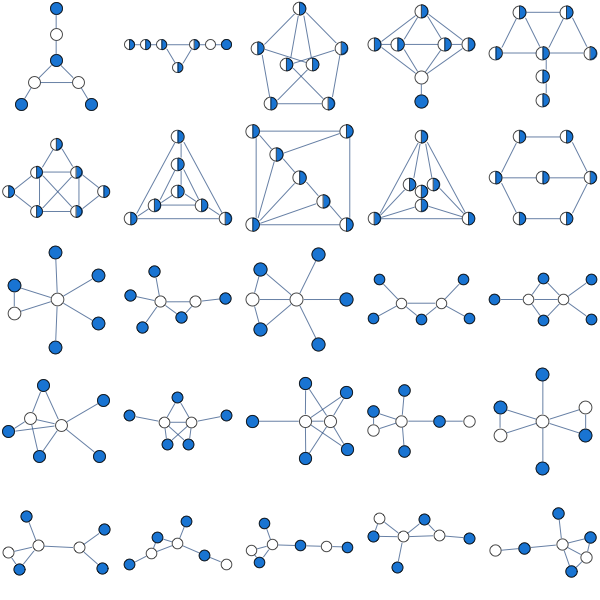A natural question to ask is when this approach works. Its guaranteed to work for bipartite graphs, but it also seems to work for many non-bipartite graphs. None of the graphs above are bipartite.

Since independent set only allows one vertex per clique, we can improve our LP by saying that sum of variables over each clique has to below 1.

Consider the following graph that our original LP has a problem with: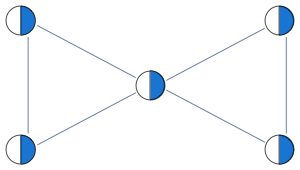Instead of having edge constraints, we formulate our LP with clique constraints as follows:

$$x_1+x_2+x_3\le 1$$
$$x_3+x_4+x_5\le 1$$

With these constraints, LP solver is able to find the integral solution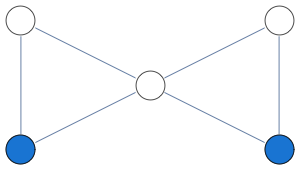In this formulation, solution of LP is no longer half-integral. For the graph below we get a mixture of 1/3 and 2/3 in the solution.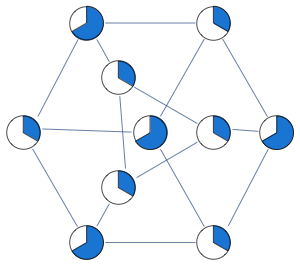Optimum of this LP is known as the "fractional chromatic number" and it is an upper bound both on the largest clique size and the largest independent set size. For the graph above, largest clique and independent set have sizes 9/3 and 12/3, whereas fractional chromatic number is 14/3.

This relaxation is tighter than previous, here are some graphs that previous LP relaxation had a problem with.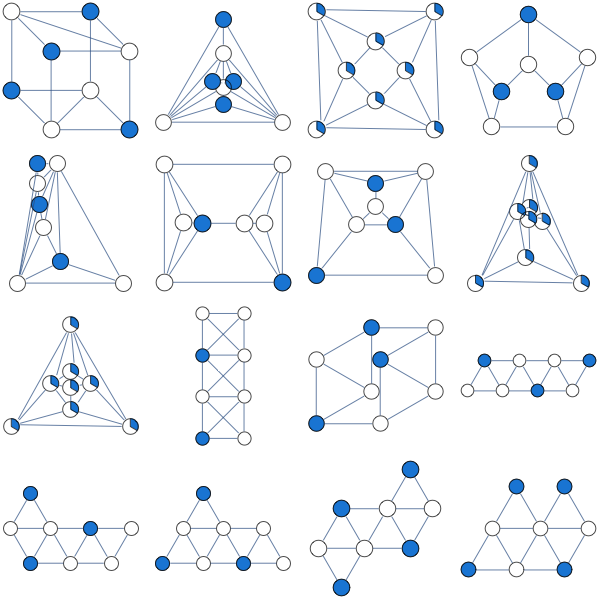This LP is guaranteed to work for perfect graphs which is a larger class of graphs than bipartite graphs. Out of 853 connected graphs over 7 vertices, 44 are bipartite while 724 are perfect.

You can improve this LP further by adding more constraints. A form of LP constraints that generalizes previous two approaches is

$$\sum_{i\in C} x_i\le \alpha(C)$$

Where $C$ is some subgraph and $\alpha(C)$ is its independence number.

You extend previous relaxation by considering subgraphs other than cliques.
Lovasz describes the following constraint classes (p.13 of "Semidefinite programs and combinatorial optimization")

1. Odd hole constraints
2. Odd anti-hole constraints
3. Alpha-critical graph constraints.

Note that adding constraints for all maximal cliques can produce a LP with exponential number of constraints.

Notebook
Packages

## Thursday, March 03, 2011

### Perils of floating point arithmetic

A recent discussion on stackoverflow brought up the issue of results of floating point arithmetic being non-reproducible

A reader asked what one could do to guarantee that result of floating point computation is always the same, and Daniel Lichtblau, a veteran developer at the kernel group of WRI replied that "it is impossible with current hardware and software"

One problem is that IEEE 754 standard is ambiguous, and different implementations of it give slightly different results. See page 250 of What Every Computer Scientist Should Know About Floating Point Arithmetic for specific examples. This means that compiling code on a different system can produce slightly different results.

Another issue is that even when you run the same computation on the same system, results can be slightly different on reruns. This has to do with how low-level optimization is scheduled.

This means that if you want your results to be reproducible, you should avoid things like testing floating point numbers for equality. John D Cook gives some more advice in Five Tips for Floating Point Programming